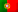Home > Technology > Computer Science > Programming > C++ > Using pointers in C / C++

# Using pointers in C / C++

For some students, handling pointers in C / C++ is hard to understand. To start with, it’s always nice to remember that everything that happens in a computer, happens in its memory. The programs are executed in memory and their data is also handled in memory. Data can be stored on disk, or on another storage device, but to a program to work with its data, it must be loaded into the computer’s RAM.

The CPU’s machine code itself uses registers to point memory addresses to execute code and to access data. When we are programming in C / C++, our variables are also stored in memory. However, sometimes, we work with variables without having to care with how they are handled in memory because, internally, it will be the compiler that will manage the memory allocation to store the variables.

On this article I’m going to make a simple introduction on how to use pointers. In future articles, I will keep talking about this subject with some more complex examples.

Let’s then start with a simple approach and a basic example of a piece of code in C:

```    int i = 5;
printf("The value of i is: %d", i);
```

Internally, the compiler will always have to allocate a memory address for the variable “i”. And this becomes more evident when we want to write a program that asks the user to enter a number:

```    int i;
printf("Enter a value for i:");
scanf("%d", &i);
printf("The value of i is: ", i);
```

Note that in the command “scanf” we wrote “&i”. The “&” symbol tells the compiler we want to pass the address of “i” and not its value. Without this notation, the command “scanf” would receive the value of the variable (that was not even initialized) and not its address! Therefore, the command will not know the memory address where to store the value entered by the user.

Let’s now see another complete example of a program that generates a random number. It’s obvious that on this example we could have used a function and it could also be shorter, but the goal is to understand how to handle with pointers when we pass arguments to a procedure or function.

```#include <stdio.h>
#include <stdlib.h>
#include <time.h>

void random(int *k) {
srand(time(NULL));     // To seed random numbers
int j = rand() % 10;   // Get a number between 0 and 9
*k = j;
}

int main() {
int i;
random(&i);
printf("i = %d", i);
return 0;
}
```

In this program, the procedure “random” receives an argument declared as “int *k” (line 5). This means that the procedure wants to receive a pointer to the memory address of a variable of the type “int” and not an integer value. Also, note how we are storing the result in the argument (line 8). If we only type “k = j” we would get an error! We have a pointer – “k” – that points to the memory address of the variable that was passed to the procedure “random”, therefore we should not change that address with some random value from a variable “j”! So, by writing “*k = j” we are telling the compiler that we want the value of “j” to be stored in the memory address pointed by “k”! Finally, the procedure call in line 13. We need to tell the compiler we want to pass the address of “i”.

And what if we want to declare “i” as a pointer? Let’s take a look on how we should write the code in the main function:

```int main() {
int *i;
random(i);
printf("i = %d", *i);
return 0;
}
```

In this case we don’t need to use “&i” when we pass the variable to the procedure “random” (line 3) because the variable already is a pointer! However, and please note that, to write the value of the variable (line 4) we can’t just enter “i”. If we do so, the program will not display the value of the variable but it’s memory address instead! So, we need to type “*i” to tell the compiler that we want to display the value stored in the pointer’s address and not the address itself.

To end with, another question that usually brings some confusion is when we work with arrays! By default, arrays are always handled as pointers in C / C++. We’ve seen above an example that asked a user to enter an integer value and we needed to type “&i” in “scanf”. Now, and what if we want to ask the user to enter a string in a chars’ array?

```    char name;